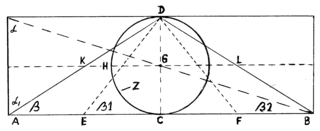## Friday, October 4, 2013

### Geometrical scheme of the UniverseGeometrical scheme of the Universe
.
DC = diameter of the circle Z =
GD = radius of the circle Z =
Angle β1 = Pyramid’s ascent = 51,85399754°
Tangent β1 = 1,273240621
CircumferenceC = (d/tang. β1) x 4
AreaA = (r²/tang. β1) x 4
Angle β = ascent of the King’s Chamber north channel = 32,48165854°
Tangent β = 0,63662031
Circumference(d/tang. β) x 2
Area
(r²/tang. β) x 2
Angle β2 = 17,65680115°
Tangent β2 = 0,318310155 = 1 : 3,14159
CircumferenceC = d/tang. β2 = AB
AreaA = r²/tang. β2

DH = r/tangβ KG = GL = AE EC = CF = FB = 1/4 AB
DC = D/tang. β = AC = CB = 1/2 AB

Tangent of the angle α (Alpha) = 3,14159 = Pi
Tangent of the angle Alpha= 1,570795 = Pi/2

The rectangle’s Area = Area of the sphere around the circle Z